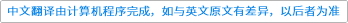Theory of Computing Systems ( IF 0.497 ) Pub Date : 2021-04-06 , DOI: 10.1007/s00224-021-10040-1
Matthieu Rosenfeld

We study the growth rate of some power-free languages. For any integer k and real β > 1, we let α(k,β) be the growth rate of the number of β-free words of a given length over the alphabet {1,2,…,k}. Shur studied the asymptotic behavior of α(k,β) for β ≥ 2 as k goes to infinity. He suggested a conjecture regarding the asymptotic behavior of α(k,β) as k goes to infinity when 1 < β < 2. He showed that for $$\frac {9}{8}\le \beta <2$$ the asymptotic upper-bound holds. We show that the asymptotic lower bound of his conjecture holds. This implies that the conjecture is true for $$\frac {9}{8}\le \beta <2$$.down
wechat
bug